Next: Simulation by ABEL Up: Nanometer Beam-Size Measurement during Previous: Introduction

# Analytic Calculation for Elliptic Beams

The equation of motion of a same-charge particle during a beam-beam collision is expressed by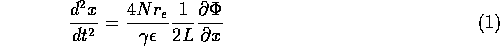(similarly for y), where N is the beam intensity, 2L the bunch length,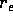the classical electron radius,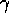the Lorentz factor of the beam energy andthe energy fraction of the particle. For a uniform charge distribution inside an elliptic cylinder of 2L long with radii a and b in the horizontal(x) and vertical(y) directions, respectively, the Coulomb potential is exactly given by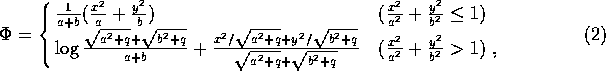where q(x,y) is the positive solution to the equation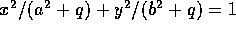. Assuming that a particle is created uniformly at a position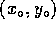with zero scattering angle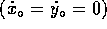, its trajectory inside the ellipsoid is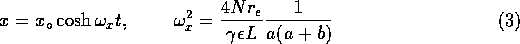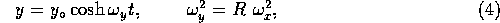where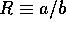is the aspect ratio of the transverse beam profile, which is equivalent to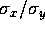for a Gaussian beam.

We first consider the azimuthal angular distribution of same-charge particles while ignoring the Coulomb field outside of the cylinder. The azimuthal angle (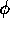) is defined by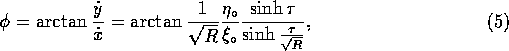where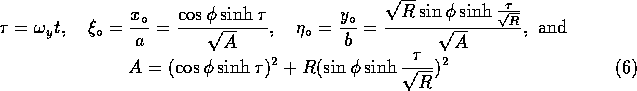under the condition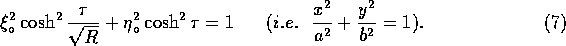The distribution function (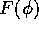), which is normalized to unity, is expressed by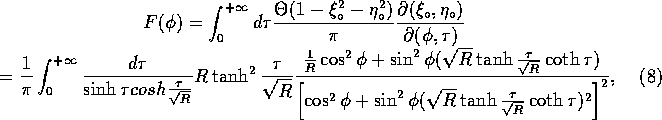where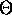is a step function. For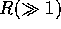and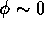(or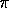), where we are interested in measuring the aspect ratio for future linear colliders,can be well approximated as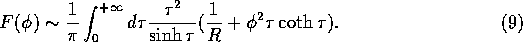As can be clearly seen in the above equations(8,9), the distribution in the angular region near to the horizontal plane is depleted for a large aspect ratio as 1/R. On the contrary, the distribution in the vertical direction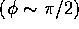has apparently no dependence on R. Therefore, we hereafter concentrate on calculations near to the horizontal plane in this section. We then estimate the effect of the Coulomb force outside of the elliptic cylinder, where the equation of motion is approximated for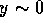and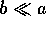, as follows: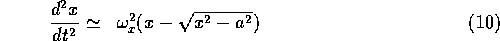and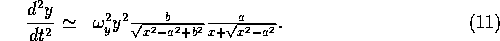Since the vertical force in Eq.(11) is very small in this region, except for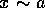, we neglect it for the moment. Integrating the x component of Eq.(10) once, for large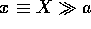we obtain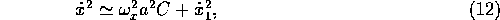where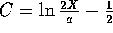and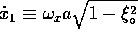, which is the x-velocity at the position (x, y) = (a, 0) . The resultant distribution function at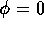is give for large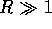by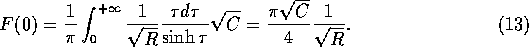We further estimated the effect of the vertical force neglected in the above calculation by numerically integrating Eqs.(10) and (11). The major effect was found to be an overall change in the magnitude by a constant factor of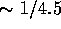. Therefore, the R dependence of F(0) becomes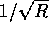instead of 1 / R ( Eq.(9)) mainly due to a horizontal force outside of the elliptic cylinder. Thus, for the case of a flat beam (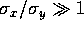), the same-charge particles are strongly deflected in the vertical direction; their azimuthal angular distribution gives us direct information about R. It should also be noticed that the particles tend to be deflected more in a direction where their creation points are displaced by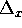and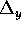, since the forces are proportional to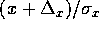and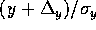, respectively, provided by differentiating Eq.(2). This gives us the sensitivity to measure the alignment between two beams, which is discussed in the next section.

The maximum deflection angle for low-energy particles can be expressed by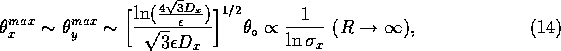where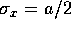,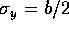,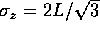and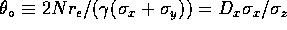. Using these relations, Gaussian beams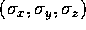can be well described in terms of variables (a,b,L) of an elliptic cylinder. Assuming that the beam intensity and the bunch length are known, the horizontal beam size can be obtained from a measurement of the maximum deflection angle, especially for a large aspect ratio, as can be clearly seen in Eq.(14).Next: Simulation by ABEL Up: Nanometer Beam-Size Measurement during Previous: Introduction

Toshiaki Tauchi
Sat Dec 21 00:34:16 JST 1996